# Texas Go Math Grade 1 Lesson 6.1 Answer Key Count On

Refer to our Texas Go Math Grade 1 Answer Key Pdf to score good marks in the exams. Test yourself by practicing the problems from Texas Go Math Grade 1 Lesson 6.1 Answer Key Count On.

## Texas Go Math Grade 1 Lesson 6.1 Answer Key Count On

Essential Question
How do you count on 1, 2, or 3?
Explanation:
counting 1 represents  as adding 1

Explore
Start at 9. How can you count on to add?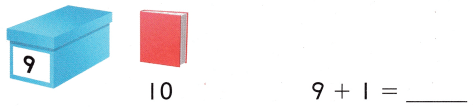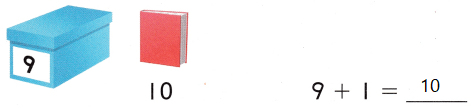Explanation:
9 + 1 = 10
counting 1 represents as adding 1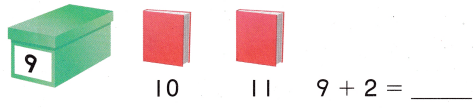Explanation:
9 + 2 = 11
counting 1 represents as adding 1Explanation:
9 + 3 = 12
counting 1 represents as adding 1

For The Teacher

• Read the problem and use the top workspace to solve. Sam has 9 books in a box. He gets 1 more. How many books does he have? Repeat for the other two workspaces, saying, He gets 2 more, and He gets 3 more.

Math Talk
Mathematical Processes
How is counting on 2 like adding 2? Explain.
Explanation:
if he counts 2 then he have 2 add 2

Model and Draw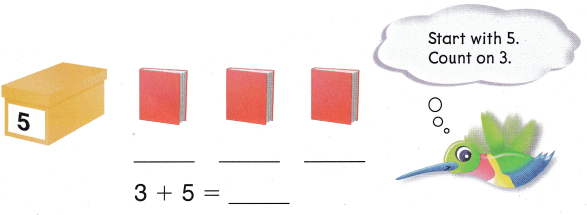Explanation:
Counting 3 represents as adding 3
Share and Show

Circle the greater addend. Drawto count on 1, 2, or 3. Write the sum.

Question 1.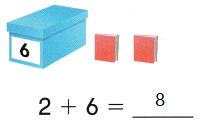Explanation:
Counting 2 represents as adding 2

Question 2.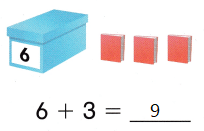Explanation:
Counting 3 represents as adding 3

Question 3.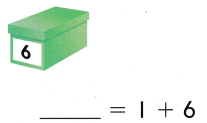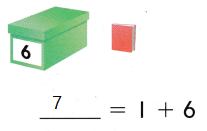Explanation:
Counting 1 represents as adding 1

Question 4.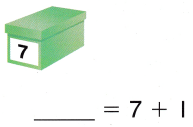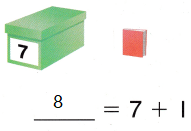Explanation:
Counting 1 represents as adding 1

Question 5.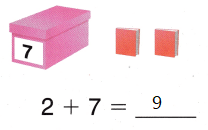Explanation:
Counting 2 represents as adding 2

Question 6.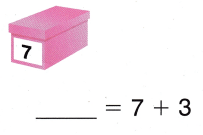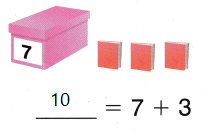Explanation:
Counting 3 represents as adding 3

Problem Solving

Count on to find the sum.

Question 7.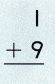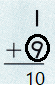Explanation:
Circled the greatest number
and started counting from that number
9 + 1 = 10

Question 8.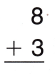Explanation:
Circled the greatest number
and started counting from that number
8 + 3 = 11

Question 9.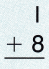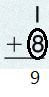Explanation:
Circled the greatest number
and started counting from that number
1 + 8 = 9

Question 10.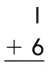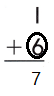Explanation:
Circled the greatest number
and started counting from that number
1 + 6 = 7

Question 11.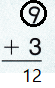Explanation:
Circled the greatest number
and started counting from that number
9 + 3 = 12

Question 12.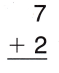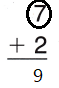Explanation:
Circled the greatest number
and started counting from that number
7 + 2 = 9

Question 13.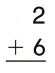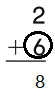Explanation:
Circled the greatest number
and started counting from that number
6 + 2 = 8

Question 14.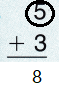Explanation:
Circled the greatest number
and started counting from that number
5 + 3 = 8

Question 15.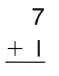Explanation:
Circled the greatest number
and started counting from that number
7 + 1 = 8

Question 16.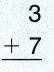Explanation:
Circled the greatest number
and started counting from that number
3 + 7 = 10

Question 17.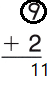Explanation:
Circled the greatest number
and started counting from that number
9 + 2 = 11

Question 18.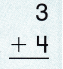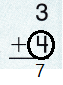Explanation:
Circled the greatest number
and started counting from that number
3 + 4 = 7

Which three numbers can you use to complete the problem? Write the addition sentence.

Question 19.
H.O.T. Jennifer has stamps. She gets more stamps. How many stamps does she have now?___ + ___ = ___ stamps
10 + 7 = 17
17 + 3 = 20
Explanation:
Started from greater number that is 10
and counted on
10 + 7 = 17
Again started from 17
17 + 3 = 20 she has 20 stamps now.

Question 20.
H.O.T. Multi-Step Adam has 6 hats. Molly has 3 hats. They stack all their hats. ___ + ___ = __ hats
Then Blake puts 2 more hats on the stock. How many hats are on the stack? ___ + ___ = __ hats
6 + 3 = 9
9 + 2 = 11
Explanation:
Started from greater number that is 6
and counted on
6 + 3 = 9 hats are on the stack
Again started from 9
9 + 2 = 11 hats are on the stack

Question 21.
Apply Ashley counts 8 sheep. Then she counts 2 more sheep. How many sheep does Ashley count?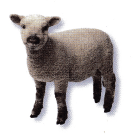(A) 10
(B) 8
(C) 12
Explanation:
Started from greater number that is 8
and counted on
8 + 2 = 10

Question 22.
Nate has 5 apples. He gets 2 more apples. How many apples does Nate have now?
(A) 8
(B) 7
(C) 6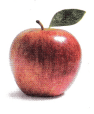Explanation:
Started from greater number that is 5
and counted on
5 + 2 = 7
7 apples that Nate have now

Question 23.
6 birds are in the tree. 2 more birds join them. How many birds are in the tree now?
(A) 9
(B) 7
(C) 8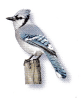Explanation:
Started from greater number that is 6
and counted on
6 + 2 = 8
8 birds are in the tree now

Question 24.
Texas Test Prep Count on to solve 3 + 8.
(A) 10
(B) 12
(C) 11
Explanation:
Started from greater number that is 8
and counted on
3 + 8 = 11

Take Home Activity

• Have your child tell you how to count on to find the sum for 6 + 3.
Explanation:
Started from greater number that is 6
and counted on
6 + 3 = 9

### Texas Go Math Grade 1 Lesson 6.1 Homework and Practice Answer Key

Circle the greater addend. Draw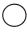to count on 1, 2, or 3. Write the sum.

Question 1.3 + 8 = ___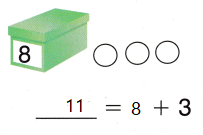Explanation:
Counting 3 represents as adding 3
3 + 8 = 11

Question 2.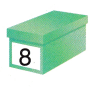__ = 8 + 1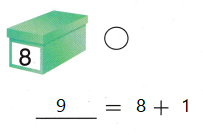Explanation:
Counting 1 represents as adding 1
8 + 1 = 9

Question 3.___ = 9 + 2Explanation:
Counting 2 represents as adding 2
11 = 9 + 2

Question 4.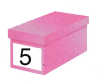5 + 3 = ___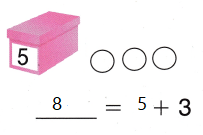Explanation:
Counting 3 represents as adding 3
5 + 3 = 8

Problem Solving.

Which three numbers can you use to complete the problem? Write the addition sentence.

Question 5.
A rabbit has 7 carrots. It gets 2 more carrots. How many carrots does the rabbit have now?
____ + ____ = ____ carrots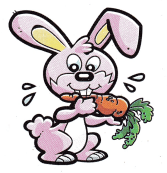7 + 2 = 9
Explanation:
Started from greater number that is 7
and counted on

Texas test Prep

Lesson Check

Question 6.
Count on to solve 1 + 4.
(A) 3
(B) 4
(C) 5
Explanation:
Started from greater number that is 4
and counted on
1 + 4 = 5

Question 7.
Char has 3 shells. She gets 7 more shells. How many shells does Char have now?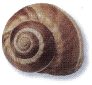(A) 4
(B) 10
(C) 11
Explanation:
3 + 7 = 10
Started from greater number that is 7
and counted on

Question 8.
Multi-Step 8 ladybugs are on a leaf. I more ladybug joins them. Then 2 more ladybugs join them. How many ladybugs are on the leaf now?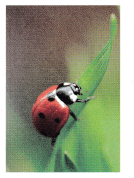(A) 11
(B) 12
(C) 10
Explanation:
8 + 1 = 9
9 + 2 = 11
11 ladybugs are on the leaf now

Question 9.
Count on to solve 3 + 9.
(A) 10
(B) 6
(C) 12